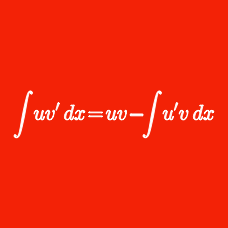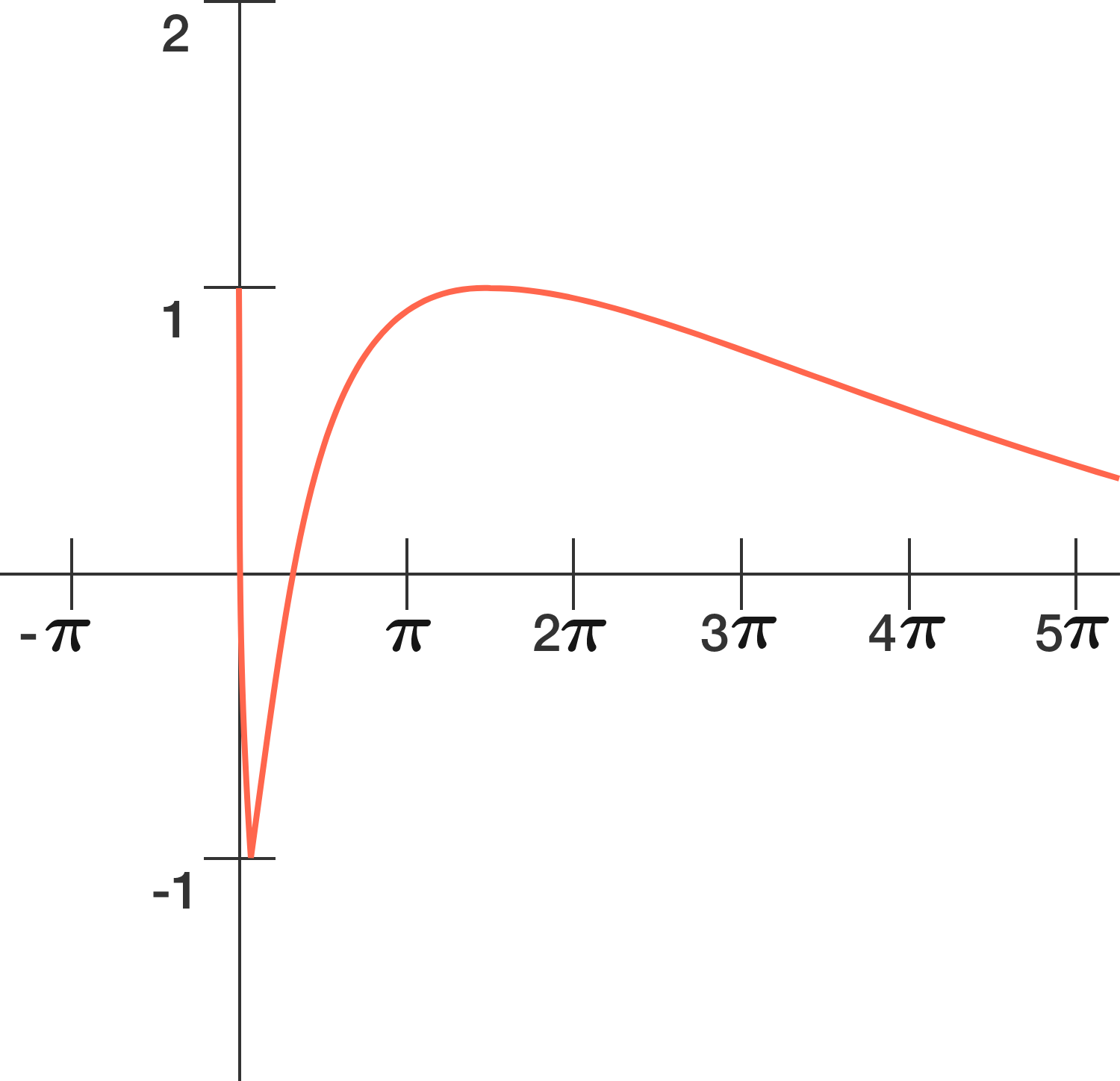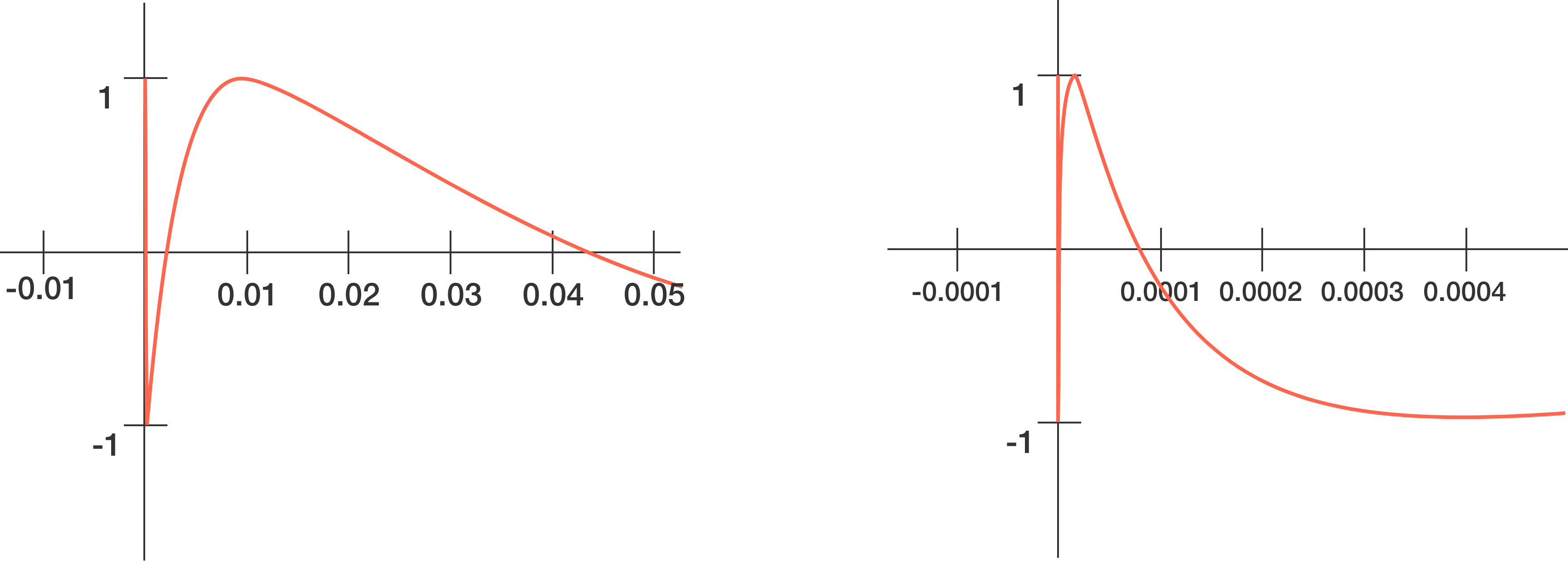Calculus

# Integration Techniques: Level 4 Challenges

$\large \int_{1}^{\infty} \dfrac {2x\{x\} - \{x\}^2} {x^2 \lfloor x \rfloor ^2} \, dx$

If the integral above can be expressed as $\dfrac{\pi^a - b} c$, where $a,b,c$ are all positive integers, find $a+b+c$.

Notations:

$\large \int_0^\infty \frac {\sin x}{xe^x} \, dx = \, ?$

$\large A = \displaystyle\int_{1}^{\infty} \left(\dfrac{1}{u^2} - \dfrac{1}{u^4}\right) \dfrac{du}{\ln u}$

Find the value of $e^A$.

Let $f(x) = x + e^x - 1$, and let $f^{-1} (x)$ denote the inverse function of $f(x)$.

Then the integral $\displaystyle \int_{e}^{1 + e^2} f^{-1} (x) \, dx$ evaluates to $k_0 + k_1 e^1 + k_2 e^2 + \cdots + k_n e^n ,$ where $k_0, k_1, \ldots, k_n$ are rational numbers and $e\approx 2.718$ is Euler's number.

If the sum $k_0 + k_1 + k_2 + \cdots + k_n$ can be expressed as $\frac{A}{B},$ where $A$ and $B$ are coprime positive integers, what is $A+B+n?$The graph of $f(x) = \sin ( \ln x )$ (as shown above) looks innocent enough to noticeably oscillate as $x$ increases. However, as $x$ approaches $0$, the oscillations grow rapidly, making $f(x + \epsilon)$ vary greatly from $f(x)$ around this region, even at very infinitesimal values of $\epsilon$.That said, $f(x)$ will cross the $x$-axis for an infinite number of times from $x=0$ to $x=1$, creating several regions of the first quadrant enclosed by the curve and the $x$-axis.

If the sum of these regions is $A$, then determine $\big\lfloor 10^5 A \big\rfloor$.

×